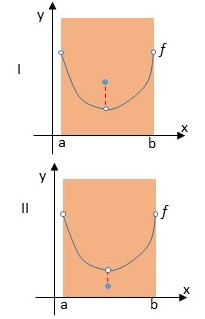# Determine from the graphs whether f has a minimum in the open interval (a, b).

## Question:

Determine from the graphs whether f has a minimum in the open interval (a, b).## Infimum and Minimum

Given a non empty set then we define k to be an infimum of A if and only if {eq}k \leq x {/eq} for all {eq}x \in A {/eq}.

A minimum of A is any number M such that M is an infimum of A and {eq}M \in A {/eq} .

So, not every infimum is a minimum but every minimum is an infimum. For an infimum to be a minimum of some set it must be a member of the set.

Also, if a set is bounded from below then the set has a infimum.

Become a Study.com member to unlock this answer! Create your account

For graph I, according to the definitions of infimum and minimum, we can notice that the function has an infimum (the little white circle on the...Minimum Values: Definition & Concept

from

Chapter 18 / Lesson 16
145K

The minimum value of a quadratic function is the low point at which the function graph has its vertex. This lesson will define minimum values and give some example problems for finding those values. A quiz will complete the lesson.